# Permittivity and Relative Permittivity or Dielectric Constant

When we place two electrically charged bodies nearby they will experience a force among them. This force may either be attractive or be repulsive depending on the nature of the charge of two bodies. Two like charged bodies repel each other and two unlike charged bodies attract each other. The value of force acting on these two nearby bodies can be formulated by Coulomb’s Law.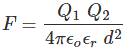The force acting between nearby electrically charged bodies depends on mainly three factors.

1. Charge of the bodies Q1 and Q2 coulombs that is the product of charges of the bodies.
2. Distance between the center of the charges i.e. d meters. The force is inversely proportional to the square of the distance d.
3. The medium in which the bodies are placed.

The role of permittivity comes in this third point. It is found that force acting between nearby same charged bodies separated by the same distance is different in different mediums. From the equation of Coulomb’s law, we find that the force acting between nearby electrically charged bodies is inversely proportional to the term εoεr. This term is called permittivity of the medium. Here, εo is known as the absolute permittivity of the vacuum and εr is the relative permittivity of the medium in which the bodies are placed.

## Relative Permittivity or Dielectric Constant

Relative Permittivity is defined as the ratio of the actual or absolute permittivity of a medium to the absolute permittivity of vacuum. If the permittivity of a medium is ε then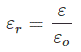This ratio is 1.0006 for air. That means relative permittivity of air is 1.0006.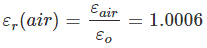The electrostatic force acting between nearby electrically charged bodies is inversely proportional to the permittivity of the medium. Hence, the relative permittivity of any medium is defined as the ratio of force acting between nearby electrically charged bodies in the vacuum to the force acting between the same bodies separated by the same distance in the medium.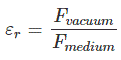We know that electric field intensity at any point in a field is defined as,From that relationship, we can establish the expression of electrical flux density (D) at that point as,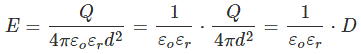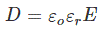From the expression of electrical field intensity and flux density we can write,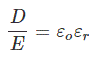It is clear that the ratio of the electric flux density to the electric field intensity at a point in the field can be defined as the permittivity of the medium at that point.

Let us take a parallel plates capacitor with effective plate area A and distance between the plates is d and the dielectric between the plates has permittivity ε. The charge accumulated in the capacitor is Q due to an applied voltage across the capacitor is V.The electric field intensity isThe flux density is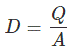The relation between flux density and electric field intensity is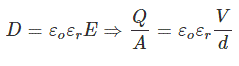Here, in the expression of capacitance, it is found that capacitance of a capacitor is directly proportional to the electric permittivity of the dielectric medium between the plates.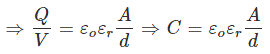Again, the expression of energy stored in the capacitor is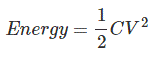From that expression, it can be concluded that the energy stored in the capacitor is directly proportional to permittivity of the medium in between the plates.So capacitance and the energy stored in the capacitor both are directly proportional to permittivity of the dielectric medium.

Hence permittivity of the medium used for making a capacitor is an essential parameter which determines the dimensions of the capacitor during designing of the capacitor.

## Absolute Permittivity

Let us define absolute permittivity. The absolute permittivity or simply permittivity of a medium can be defined as the property of the medium which determines certain electric field intensity at a point in the field creates how much flux density at that point. We will show it later in this article. The absolute permittivity of any medium can be represented by the product of two terms one is absolute permittivity of vacuum and relative permittivity of the medium. This is for simplifying the calculations based on permittivity. The absolute permittivity of vacuum is taken as the base value of the permittivity. The relative permittivity of a medium is how many times the permittivity of the medium greater than the absolute permittivity of vacuum.

## Unit of Permittivity

#### Unit of Absolute Permittivity

From the equation of Coulomb’s law, we can write the expression of permittivity as,From the expression of permittivity, as shown above, we can determine the unit of permittivity as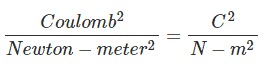#### Another Unit of Absolute Permittivity

The relationship between capacitance and permittivity of dielectric medium of a capacitor can be expressed as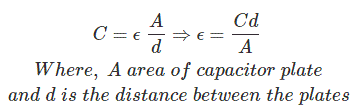From this expression, the unit of permittivity comes as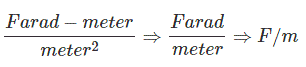#### Unit of Relative Permittivity

As relative permittivity is the ratio of absolute permittivity of a medium to the absolute permittivity of vacuum this is unit less quantity.

### Permittivity of Free Space

The permittivity of free space is also called vacuum permittivity. The value of permittivity of free space is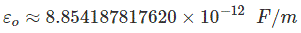Want To Learn Faster? 🎓
Get electrical articles delivered to your inbox every week.
No credit card required—it’s 100% free.